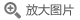## 偏微分方程(第4版影印版)(英文版)

• 定价： ￥79
• ISBN：9787519255800
• 开 本：16开 平装
• 作者：(美)F.约翰
• 立即节省：
• 2019-07-01 第1版
• 2019-07-01 第1次印刷### 导语### 内容提要本书是一部非常优秀的介绍偏微分方程的入门书籍，可以作为研究生阶段学习的基石。本书详尽地介绍了偏微分方程理论的重要方面，并从数学分析的角度做了进一步的探讨。本书是第4版，增加了全新的一章讲述无解线性方程的Lewy例子。

### 目录

Chapter 1  The Single First-Order Equation
1.Introduction
2.Examples
3.Analytic Solution and Approximation Methods in a Simple Example
Problems
4.Quasi-linear Equations
5.The Cauchy Problem for the Quasi-linear Equation
6.Examples
Problems
7.The General First-Order Equation for a Function of Two Variables
8.The Cauchy Problem
9.Solutions Generated as Envelopes
Problems
Chapter 2  Second-Order Equations: Hyperbolic Equations for Functions of Two Independent Variables
1.Characteristics for Linear and Quasi-linear Second-order Equations
2.Propagation of Singularities
3.The Linear Second-Order Equation
Problems
4.The One-Dimensional Wave Equation
Problems
5.Systems of First-Order Equations
6.A Quasi-linear System and Simple Waves
Problem
Chapter 3  Characteristic Manifolds and the Cauchy Problem
1.Notation of Laurent Schwartz
Problems
2.The Cauchy Problem
Problems
3.Real Analytic Functions and the Cauchy-Kowalevski Theorem
(a)  Multiple infinite series
Problems
(b)  Real analytic functions
Problems
(c)  Analytic and real analytic functions
Problems
(d)  The proof of the Cauchy-Kowalevski theorem
Problems
4.The Lagrange-Green Identity
5. The Uniqueness Theorem of Holmgren
Problems
6.Distribution Solutions
Problems
Chapter 4  The Laplace Equation
1.Green's Identity, Fundamental Solutions, and Poisson's Equation
Problems
2.The Maximum Principle
Problems
3.The Dirichlet Problem, Green's Function, and Poisson's Formula
Problems
4.Proof of Existence of Solutions for the Dirichlet Problem Using Subharmonic Functions ("Perron's Method")
Problems
5.Solution of the Dirichlet Problem by Hilbert-Space Methods
Problems
Chapter 5  Hyperbolic Equations in Higher Dimensions
1.The Wave Equation in n-Dimensional Space
(a)  The method of spherical means
Problems
Problems
(c)  Duhamers principle and the general Cauchy problem
Problem
(d)  Initial-boundary-value problems ("Mixed" problems)
Problems
2.Higher-Order Hyperbolic Equations with Constant Coefficients
(a)  Standard form of the initial-value problem
Problem
(b) Solution by Fourier transformation
Problems
(c)  Solution of a mixed problem by Fourier transformation
(d) The method of plane waves
Problems
3.Symmetric Hyperbolic Systems
(a) The basic energy inequality
Problems
(b) Existence of solutions by the method of finite differences
Problems
(c)  Existence of solutions by the method of approximation by analytic functions (Method of Schauder)
Chapter 6  Higher-Order Elliptic Equations with Constant Coefficients
1.The Fundamental Solution for Odd n
Problems
2. The Dirichlet Problem
Problems
3.More on the Hilbert Space Hg and the Assumption of Boundary Values in the Dirichlet Problem
Problems
Chapter 7  Parabolic Equations
1.The Heat Equation
(a)  The initial-value problem
Problems
(b) Maximum principle, uniqueness, and regularity
Problem
(c)  A mixed problem
Problems
(d)  Non-negative solutions
Problems
2.The Initial-Value Problem for General Second-Order Linear Parabolic Equations
(a)  The method of finite differences and the maximum principle
(b)  Existence of solutions of the initial-value problem
Problems
Chapter 8  H.Lewy's Example of a Linear Equation without Solutions
Problems
Bibliography
Glossary
Index